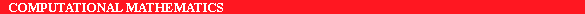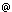ERCIM News No.22 - July 1995 - CNR

# Parallel Linear Algebra Algorithms and Applications at IMC-CNR

by Bruno Codenotti and Mauro Leoncini

Numerical Linear Algebra methods provide the computational primitives to solve many important problems in applied sciences. For this reason, the exploitation of the power of parallel machines by algorithms that implement these methods has become a core area of research in numerical analysis.

At IMC-CNR we are involved in a number of national and international projects whose scope ranges from the understanding of the fundamental aspects of parallel computing and parallel complexity, to the development of parallel algorithms for specific (parallel or distributed) architectures which solve certain application problems.

With respect to applications, we focus primarily on parallel algorithms that solve computational problems of importance in the following fields:

• Digital filtering
• Image processing
• Volume rendering

We are now developing parallel algorithms that compute certain discrete transforms. These algorithms are being implemented on an NCUBE2, a distributed memory machine with 128 processors physically laid at the vertex of an interconnection network with hypercube topology.

In particular we concentrate on

• Fast Fourier Transform algorithms and their application to the analyis and filtering of digital signals;
• Hough Transform algorithms, which are particularly useful for image analysis in the presence of rectilinear borders;
• wavelets and applications for factor scale detection in 2 dimensional images.

We are also interested in the development of fast and efficient algorithms which are well-known tools for solving general linear algebraic problems (without concentrating specifically on the problems). In particular, we have developed parallel algorithms for solving tridiagonal and block tridiagonal systems of linear equations. These algorithms have been explicitly designed to take advantage of the two level parallelism provided by today's supercomputers: the outer parallelism is provided by the availability of many processors, and the inner parallelism by the presence of vector units inside a processor. We have implemented and tested our algorithms on a CM5 with 32 processors (and a total of 128 vector units), obtaining very good running times.

E-mail: leonciniiei.pi.cnr.it